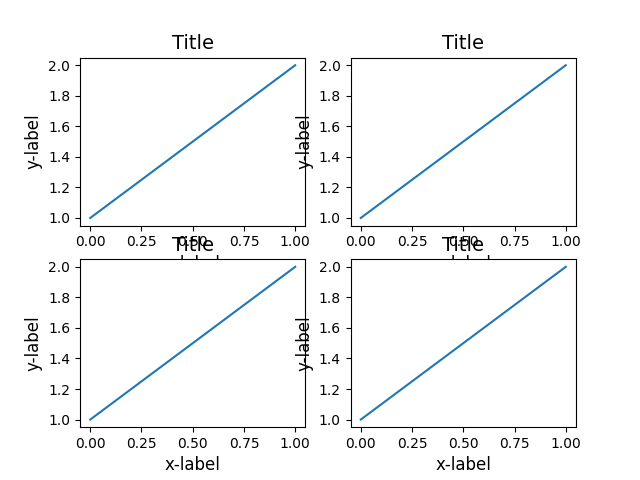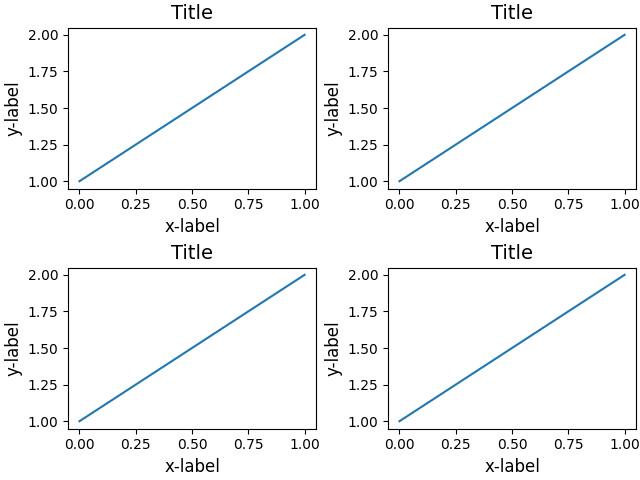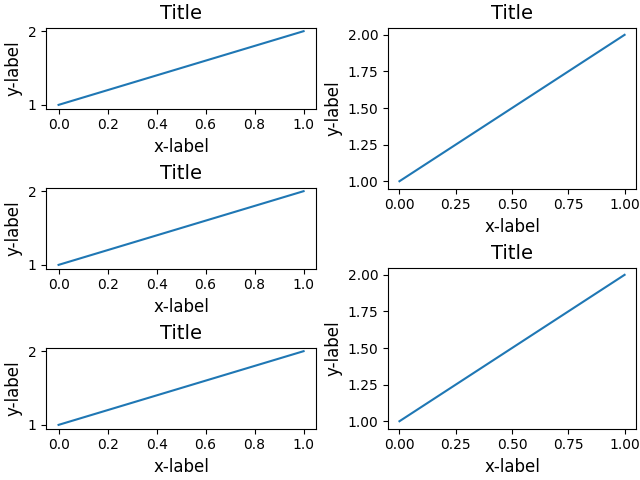# Resizing axes with constrained layout#

Constrained layout attempts to resize subplots in a figure so that there are no overlaps between axes objects and labels on the axes.

See Constrained Layout Guide for more details and Tight Layout guide for an alternative.

import matplotlib.pyplot as plt

def example_plot(ax):
ax.plot([1, 2])
ax.set_xlabel('x-label', fontsize=12)
ax.set_ylabel('y-label', fontsize=12)
ax.set_title('Title', fontsize=14)


If we don't use constrained layout, then labels overlap the axes

fig, axs = plt.subplots(nrows=2, ncols=2, layout=None)

for ax in axs.flat:
example_plot(ax)adding layout='constrained' automatically adjusts.

fig, axs = plt.subplots(nrows=2, ncols=2, layout='constrained')

for ax in axs.flat:
example_plot(ax)Below is a more complicated example using nested gridspecs.

fig = plt.figure(layout='constrained')

import matplotlib.gridspec as gridspec

gs0 = gridspec.GridSpec(1, 2, figure=fig)

gs1 = gridspec.GridSpecFromSubplotSpec(3, 1, subplot_spec=gs0)
for n in range(3):
example_plot(ax)

gs2 = gridspec.GridSpecFromSubplotSpec(2, 1, subplot_spec=gs0)
for n in range(2):